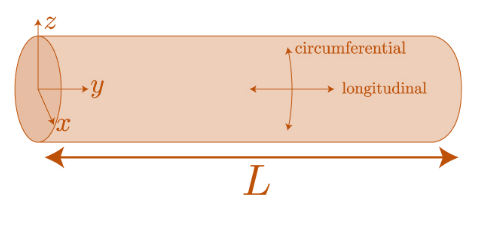# internal pressure on a thin pipe causes a stress . this stress is known as: A. LONGITUDINAL STRESSB. CENTRIFUGAL STRESS C.HOOP STRESSD.TANGENTIAL STRESS

Question

internal pressure on a thin pipe causes a stress . this stress is known as:

A. LONGITUDINAL STRESS

B. CENTRIFUGAL STRESS

C.HOOP STRESS

D.TANGENTIAL STRESS

check_circle

Step 1

Longitudinal stress:  It is defined as the force acting along the longitudinal direction or in the axial direction of the pipe.

Step 2

Hoop stress/Tangential stress:

It is defined as the force acting perpendicular both to the radius and axis of the pipe along each point of circumference in both the directions. It is defined as the normal stress along tangential direction.

As the length of the pipe is more as compared to the end diameter so, the hoop stress has more value as compared to longitudinal stress. Hence, the correct answer is hoop stress.Step 3

Centrifugal stress: This type of stress does...

### Want to see the full answer?

See Solution

#### Want to see this answer and more?

Solutions are written by subject experts who are available 24/7. Questions are typically answered within 1 hour.*

See Solution
*Response times may vary by subject and question.
Tagged in

### Mechanical Engineering